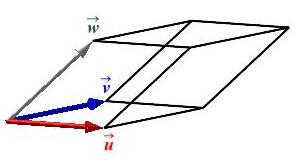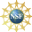# 5. Cross Product

## 2. Geometric Interpretation of Triple Products

The expression $$\vec u\cdot\vec v\times\vec w$$ is the algebraic formula for the triple product. Since the triple product is a scalar, the geometrical interpretation only needs to explain one number. However, we still give the interpretation in two pieces, the absolute value and the sign of the triple product.

The absolute value of the triple product $$\vec u\cdot\vec v\times\vec w$$ is the volume of the parallelepiped with adjacent edges $$\vec u$$, $$\vec v$$ and $$\vec w$$: $V=|\vec u\cdot\vec v\times\vec w|$

This was proven on the previous page.The sign of the triple product determines the handedness of the $$3$$ vectors, as follows:

The vectors $$\vec u$$ and $$\vec v$$ span a plane and the vector $$\vec u\times\vec v$$ points to one side of that plane determined by the right hand rule. Based on this, we have the following two definitions and a proposition which give the geometric interpretation of the sign of the triple product:

The vectors $$\vec u$$, $$\vec v$$ and $$\vec w$$ are called a right handed system of vectors if: $\vec u\times\vec v\cdot\vec w \gt 0$ Equivalently, $$\vec u$$, $$\vec v$$, $$\vec w$$ is a right handed system if $$\vec w$$ forms an acute angle with $$\vec u\times\vec v$$; i.e. $$\vec w$$ is on the same side of the $$\vec u\vec v$$-plane as $$\vec u\times\vec v$$.

The vectors $$\vec u$$, $$\vec v$$ and $$\vec w$$ are called a left handed system of vectors if: $\vec u\times\vec v\cdot\vec w \lt 0$ Equivalently, $$\vec u$$, $$\vec v$$, $$\vec w$$ is a left handed system if $$\vec w$$ forms an obtuse angle with $$\vec u\times\vec v$$; i.e. $$\vec w$$ is on the opposite side of the $$\vec u\vec v$$-plane as $$\vec u\times\vec v$$.

The vectors $$\vec u$$, $$\vec v$$ and $$\vec w$$ are co-planar if   $$\vec u\times\vec v\cdot\vec w=0$$

The equation, $$\vec u\times\vec v\cdot\vec w=0$$, says $$\vec w$$ is perpendicular to $$\vec u\times\vec v$$. So $$\vec w$$ lies in the same plane as $$\vec u$$ and $$\vec v$$.

We summarize:

The sign of the triple product $$\vec u\cdot\vec v\times\vec w$$ determines that $$\vec u$$, $$\vec v$$ and $$\vec w$$ are

1. a right handed system   if   $$\vec u\times\vec v\cdot\vec w \gt 0$$.
2. a left handed system    if   $$\vec u\times\vec v\cdot\vec w \lt 0$$.
3. co-planar           if   $$\vec u\times\vec v\cdot\vec w=0$$.

Show the vectors $$\hat\imath$$, $$\hat\jmath$$ and $$\hat k$$ are a right handed system of vectors. Show the vectors $$\hat k$$, $$\hat\jmath$$ and $$\hat\imath$$ are a left handed system of vectors.

We simply compute \begin{aligned} \hat\imath\times\hat\jmath\cdot\hat k &=\hat k\cdot\hat k=1 \gt 0 &&\Longrightarrow \quad \hat\imath, \hat\jmath, \hat k\,\text{is right handed.} \\ \hat k\times\hat\jmath\cdot\hat\imath &=-\hat\imath\cdot\hat\imath=-1 \lt 0 &&\Longrightarrow \quad \hat k, \hat\jmath, \hat\imath\,\text{is left handed.} \end{aligned}

Determine whether each of the following systems is right handed, left handed or co-planar.

1. $$\vec u=\left\langle 2,1,3\right\rangle$$, $$\vec v=\left\langle 2,0,1\right\rangle$$, $$\vec w=\left\langle 4,-2,1\right\rangle$$.

Left-handed

$$\vec u\times\vec v\cdot\vec w= \begin{vmatrix} 2 & 1 & 3 \\ 2 & 0 & 1 \\ 4 & -2 & 1 \end{vmatrix} =-6\qquad$$ Therefore, left-handed

2. $$\vec a=\left\langle 4,1,-2\right\rangle$$, $$\vec b=\left\langle 2,3,0\right\rangle$$, $$\vec c=\left\langle -1,3,2\right\rangle$$.

Right-handed

$$\vec a\times\vec b\cdot\vec c= \begin{vmatrix} 4 & 1 & -2 \\ 2 & 3 & 0 \\ -1 & 3 & 2 \end{vmatrix} =2\qquad$$ Therefore, right-handed

3. $$\vec p=\left\langle -3,2,4\right\rangle$$, $$\vec q=\left\langle 2,1,-3\right\rangle$$, $$\vec r=\left\langle 1,4,-2\right\rangle$$.

Co-planar

$$\vec p\times\vec q\cdot\vec r= \begin{vmatrix} -3 & 2 & 4 \\ 2 & 1 & -3 \\ 1 & 4 & -2 \end{vmatrix} =0\qquad$$ Therefore, co-planar

Supported in part by NSF Grant #1123255